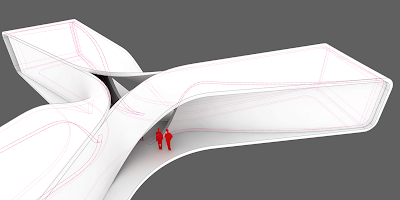## 18 November 2012

I started from a tutorial of the structure of the Gradient Descent algorithm wrote by Woo Jae and I rewrote the entire code from VB language to a VbRhinoscript language. I wrote this version of code one year ago but in these days I had the time to debug and post it.
Testing the speed of each codes I can admit that the VB version is faster than the VbRH, also the VB code is very short but I wrote the VBRH version just for exercise and to customize the code adding other tasks like  the type of algorithm ( normal o rotated) the distance factor of the span of the drain vector, layers subdivivded for the components of the algorithm ( construction vectors, drain vectors, numbered text dots for all curves) and a counter that shows the progress of the code in real time.

Option Explicit
'Script written by davide del giudice
'Script version Tuesday, 13 December 2011 15:39:13

rhino.MessageBox "make sure that your object has flipped normal and any points with negative Z"
Dim arrpt:arrpt= rhino.GetObjects ( "select points", 1)
Dim surf:surf= rhino.GetObject ("select surface",8)

Dim arrNames(1)
arrNames(0) = "normal"
arrNames(1) = "rotated"

Dim strCmd : strCmd = rhino.GetString("How do u want your algorithm? ",, arrNames )
Dim distancefactor:distancefactor= Rhino.GetInteger("Enter the distance factor (it depends to the object dimensions)", 10, 0,100)

rhino.HideObjects arrpt
rhino.HideObject surf

Dim ptcounter:ptcounter= Ubound(arrpt)

Dim pt

For Each pt In arrpt

Dim count
count= count+1
Call outputpoinF(pt,surf,distancefactor,strCmd,count)
rhino.print ("script at" & " " & 100-(round((((ptcounter-count)/ptcounter)*100),0)) & "%" & " " &"completed")

Next

End Sub

Function outputpoinF(pt,surf,distancefactor,strCmd,count)

Rhino.EnableRedraw False

rhino.CurrentLayer "vectors"
Dim arrpt:arrpt= rhino.PointCoordinates (pt)

If arrpt(2)<0 .1=".1" font="font" then="then">
Dim arrobjects4,strobject4,arrpoints4
arrObjects4 = Rhino.NormalObjects
strobject4=rhino.JoinCurves (arrobjects4)
arrPoints4 = Rhino.CurveEditPoints(strObject4(0))
rhino.CurrentLayer "interpcurves"
rhino.Command  "_SelAll _Hide"
Exit Function

End If

Dim arrParam:arrParam=rhino.SurfaceClosestPoint (surf, arrpt)
Dim arrptUV: arrptUV = Rhino.EvaluateSurface(surf, arrParam)
rhino.HideObject outputpoint
Dim arrnormal:arrnormal= Rhino.SurfaceNormal (surf, arrParam)
Dim zpoint2:zpoint2= (array(arrptUV(0),arrptUV(1),arrptUV(2)+1))
Dim  arrVector2 : arrVector2= Rhino.VectorCreate (arrptUV , zpoint2)

'--------------------
Dim arrowz:arrowz=rhino.CurveArrows (linez,2)

rhino.HideObject linez
rhino.HideObject arrowz
'--------------------

'--------------------
Dim arrown:arrown= rhino.CurveArrows (linenormal,2)
rhino.HideObject linenormal
rhino.HideObject arrown

'--------------------

Dim veccompare:veccompare=Rhino.VectorCompare (arrnormal, arrVector2)
If veccompare=0 Then
Exit Function
End If

Dim vectorcross:vectorcross= Rhino.VectorCrossProduct (arrnormal, arrVector2)
Dim endpt2:endpt2= rhino.vectoradd ( arrptUV, vectorcross)

'--------------------
Dim distcross:distcross= rhino.Distance (arrptUV,endpt2)

If distcross =0 Then

Exit Function
End If

Dim arrowcross:arrowcross=rhino.CurveArrows (linecross,2)
rhino.HideObject linecross
rhino.HideObject arrowcross
'--------------------

Dim drainvector

If strCmd = "normal" Then
'----------------------
drainvector= Rhino.VectorRotate ( vectorcross, -90, arrVector2)
ElseIf strCmd = "rotated" Then
'----------------------
drainvector= Rhino.VectorRotate ( vectorcross, (-10*(50)), arrVector2)

End If

outputpoint= Rhino.MoveObject (outputpoint, array((drainvector(0))*distancefactor,(drainvector(1))*distancefactor, (drainvector(2))*distancefactor))
Dim arrpt2:arrpt2= rhino.PointCoordinates ( outputpoint)
Dim arrParam2:arrParam2=rhino.SurfaceClosestPoint (surf, arrpt2)
Dim arrptUV2: arrptUV2 = Rhino.EvaluateSurface(surf, arrParam2)

rhino.CurrentLayer "outputpoint2"

rhino.HideObject outputpoint2
Dim outputpointF

If  arrptUV2(2)=  arrptUV(2) Then
Dim arrobjects2,strobject2,arrpoints2
arrObjects2 = Rhino.NormalObjects
strobject2=rhino.JoinCurves (arrobjects2)
arrPoints2 = Rhino.CurveEditPoints(strObject2(0))
rhino.CurrentLayer "interpcurves"
rhino.Command  "_SelAll _Hide"
Exit Function
Else

rhino.CurrentLayer "construction"
'--------------------
Dim arrowline1:arrowline1=rhino.CurveArrows (line1,2)
rhino.HideObject arrowline1
'--------------------
Dim curveL:curveL= rhino.CurveLength (line1)

If arrptUV2(2) >= arrptUV(2) Then
outputpointF= False
Else
'outputpointF= True

If  curveL < 1 Then
Dim arrobjects,strobject,arrpoints
arrObjects = Rhino.NormalObjects
strobject=rhino.JoinCurves (arrobjects)
arrPoints = Rhino.CurveEditPoints(strObject(0))
rhino.CurrentLayer "interpcurves"

Dim endptintcrv:endptintcrv= rhino.CurveEndPoint (intcrv)
Call rhino.ObjectColor ( textdotpt, RGB(127,255,count+10))

rhino.Command  "_SelAll _Hide"
Exit Function
End If
End If
End If

Call outputpoinF(outputpoint2,surf,distancefactor,strCmd,count)
Rhino.EnableRedraw True

End Function

original Woo Jae's VB code with a little variation on the drain vector, download the GH code from Co-de-iT grasshopper code page:

## 12 April 2012

### GRASSHOPPER // Clever Skin workshop ROMAGrasshopper// Clever skin workshop at Spazio 5 Rome, via Crescenzio 99/c May 5 to May 6 2012, more info at: http://www.co-de-it.com/wordpress/cognition-workshop-series-rome.html

## 19 March 2012

### RHINOCEROS workshop_ROME3d model inspired by UNStudio the changing room pavilion

Rhinoceros // blend geometries workshop: if you're interested contact us at cognitiowsATgmail.com, training places still available, info at: http://www.co-de-it.com/wordpress/cognition-workshop-series-rome.html